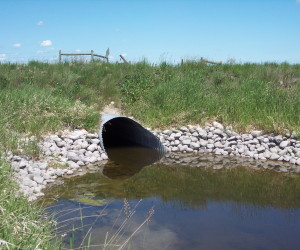# How to Calculate Hydraulic RadiusHydraulic radius is used in various engineering applications, particularly in the calculation of pipe and channel flow. It is defined as the flow area divided by the wetted perimeter.$r_h = \frac{Flow Area}{Wetted Perimeter}$

## Flow Area

This is the cross-sectional flow area of the channel or pipe. When a pipe is flowing under pressure, this is the full internal area of the pipe.

## Wetted Perimeter

This is the length of the line representing the interface between the fluid and the pipe or channel. It does not include the free surface length.

## Example – Circular culvert flowing full

A circular culvert flowing completely full has a flow area of πr2 and a wetted perimeter of 2πr, meaning the hydraulic radius is:$r_h = \frac{\pi r^{2}}{2\pi r} = \frac{r}{2}$

## Interpretation

The greater the hydraulic radius, the greater the efficiency of the pipe (or channel) and the greater the volume of flow it can carry.

This site uses Akismet to reduce spam. Learn how your comment data is processed.